﻿ 切削废液处理设备的设计研究 Design and Research of Cutting Waste Treatment Equipment

Instrumentation and Equipments
Vol. 06  No. 03 ( 2018 ), Article ID: 27050 , 7 pages
10.12677/IaE.2018.63019

Design and Research of Cutting Waste Treatment Equipment

Jing Liao, Tao Zhang*, Shuo Sun, Zongyang Ji, Zhe Xue, Chen Shen

Wuxi Institute of Technology, Wuxi Jiangsu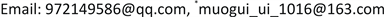Received: Sep. 6th, 2018; accepted: Sep. 23rd, 2018; published: Sep. 30th, 2018ABSTRACT

This paper designs a set of cutting waste liquid processing equipment, including slow flow devices and oil-water separation devices, based on the principle of different oil-water density. According to the shallow pools principle and stokes law, the box structure of the equipment is reasonably designed, and one device can achieve a processing efficiency of 17 L/min. This set of equipment has the characteristics of simple structure, convenient installation and handling, and is mainly suitable for small and medium-sized enterprises.

Keywords:Cutting Waste Liquid, Oil-Water Density, SmallCopyright © 2018 by authors and Hans Publishers Inc.1. 引言

2. 切削废液处理设备的结构设计

2.1. 切削废液处理器的设计要求

2.2. 工作原理

2.3. 主要部件

2.3.1. 缓流装置的设计1.隔膜泵；2.过滤器；3.缓流器；4.导管；5.吸油器；6.集油箱；7.集水箱；8.升降管；9.沉淀池

Figure 1. Structure diagram of cutting waste liquid processor

2.3.2. 箱体的设计

2.3.3. 螺纹管的设计

3. 沉淀池的设计

3.1. 粘度

3.2. 切削废液受力分析

( ${\rho }_{s}$ 为颗粒密度，d为颗粒直径， $\rho$ 为流体密度)因为 $G-{F}_{b}-{F}_{f}=ma$ ，所以 $\frac{\text{π}}{6}{d}^{3}{\rho }_{s}g-\frac{\text{π}}{6}{d}^{3}\rho g-\xi \cdot \frac{\text{π}}{4}{d}^{2}\cdot \frac{\rho {v}^{2}}{2}=\frac{\text{π}}{6}{d}^{3}{\rho }_{s}a$ ( $\xi$ 为阻力系数)。

$\frac{\text{π}}{6}{d}^{3}{\rho }_{s}g-\frac{\text{π}}{6}{d}^{3}\rho g-\xi \cdot \frac{\text{π}}{4}{d}^{2}\cdot \frac{\rho {v}^{2}}{2}=0$

3.3. 斯托克斯定律

$f=6\text{π}\eta vr$ ( $\eta$ 为粘滞系数，v为球形物体速度，r为球形物体半径)

$\mu$ 为流体粘度，r为颗粒半径，g为重力加速度。该方程可用于解释沉积在盆地的颗粒粒度分布，以及不同形状，密度和半径的颗粒混合在一起的情况。

3.4. 浅池原理

20世纪初，哈真提出了浅池理论：设斜管沉淀池长度为L，颗粒沉速为 ${u}_{0}$ ，在理想状态下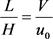。可以得出L与V的值不变时，沉淀池池身越浅，可被去除的悬浮物颗粒就越小。若用水平隔板将H分成3层，每层层深为 $\frac{H}{3}$ ，在 ${u}_{0}$ 与V不变的情况下，只需 $\frac{H}{3}$ 就可以将沉速为 ${u}_{0}$ 的颗粒去除。即沉淀池的总容积可以减少到原来的 $\frac{1}{3}$ 。反的来说，如果L不变，池深为 $\frac{H}{3}$ ，那么水流速度可增加到3V，也可以将沉速为 ${u}_{0}$ 的颗粒去除。由此可以得出：将沉淀池池身分成n层，那么处理效率就能提高n倍。

3.5. 数值计算

4. 效率计算

4.1. 流体的连续性方程

$\frac{\partial \rho }{\partial t}+\frac{\partial \left(\rho u\right)}{\partial x}+\frac{\partial \left(\rho v\right)}{\partial y}+\frac{\partial \left(\rho w\right)}{\partial z}=0$ ，这个方程研究的是控制体，称为流体力学中的连续性方程。在本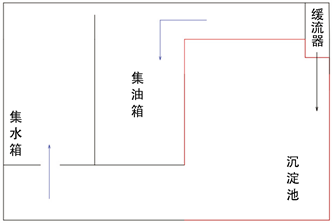Figure 2. Internal structure of box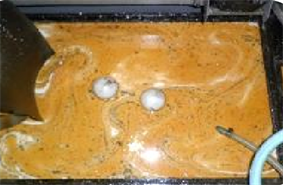Figure 3. Before processing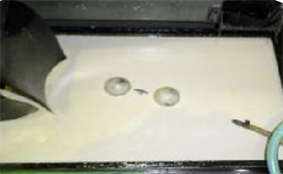Figure 4. After processing

4.2. 工作效率

5. 处理效果

6. 结论

Design and Research of Cutting Waste Treatment Equipment[J]. 仪器与设备, 2018, 06(03): 123-129. https://doi.org/10.12677/IaE.2018.63019

1. 1. 刘镇昌. 切削液技术[M]. 机械工业出版社, 2009.

2. 2. 丁祖荣. 流体力学(上册) (第二版) [M]. 北京: 高等教育出版社, 2013.

3. 3. 刘国勇. 流体力学数值方法[M]. 北京: 冶金工业出版社, 2016.

4. 4. 张维佳. 流体力学[M]. 中国建筑工业出版社, 2011.

5. 5. 齐鄂荣, 曾玉红. 工程流体力学(第2版) [M]. 武汉大学出版社, 2012.

6. 6. 滑春雨. 乳化液废水处理应用实例[J]. 露天采矿技术, 2006(4): 41-44.

7. 7. 吕兆歧, 谢泉. 油品研究与应用指南[M]. 中国石化出版社, 1997: 267.

NOTES

*通讯作者。